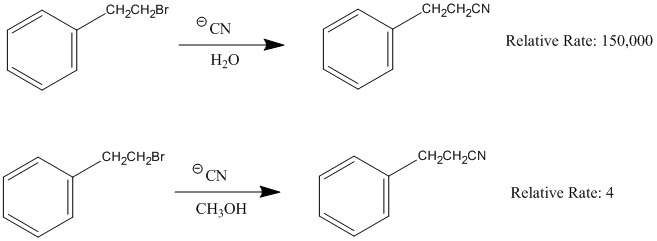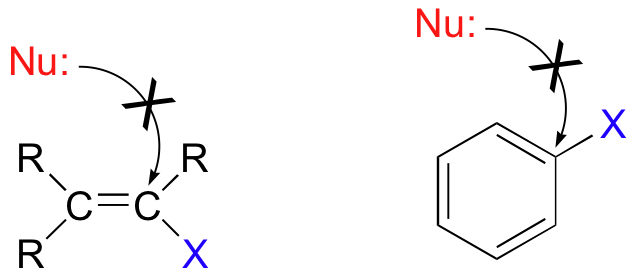Skip to main content

# 11.6: The SN1 Reaction

Objectives

After completing this section, you should be able to

1. write an expression relating reaction rate and reactant concentration for a first-order reaction.
2. compare the kinetics of SN1 and SN2 reactions.
3. identify the rate-limiting step for a reaction, given the reaction energy diagram.
4. sketch a reaction energy diagram for a reaction, given the mechanism and sufficient information to identify the rate-limiting step.
5. write the mechanism of a typical SN1 reaction, and discuss the important features of the mechanism.
6. discuss the stereochemistry of an SN1 reaction, and explain why a racemic mixture is expected when substitution takes place at the chiral carbon atom of an optically pure substrate.
7. explain why unimolecular nucleophilic substitution at the chiral carbon atom of an optically pure substrate does not result in complete racemization.
8. compare the stereochemical consequences of the SN1 mechanism with those of the SN2 mechanism.

Key Terms

Make certain that you can define, and use in context, the key terms below.

• first-order reaction
• rate-limiting step
• SN1

Study Notes

You should recognize that certain compounds (notably tertiary alkyl halides) which react very slowly by the SN2 mechanism can undergo rapid nucleophilic substitution by an alternative mechanism.

The abbreviation SN1 refers to “unimolecular nucleophilic substitution.” In a first-order reaction, the rate of the reaction depends on the concentration of only one of the reactants. Thus, when an alkyl halide reacts by an SN1 mechanism, the rate of reaction is dependent on the concentration of the alkyl halide, but is independent of the concentration of the attacking nucleophile.

Note: In many textbooks the “rate-limiting step” is called the “rate-determining step.”

Racemization problems can be a potential source of confusion. Most students entering an introductory organic chemistry course have a reasonable background in mathematics, and feel comfortable if they have a formula or equation they can use in this type of situation. Thus, we recommend that you consider the following approach.

1. In a given mixture of enantiomers, let x = the fraction of the (+)-enantiomer, and 1 − x = the fraction of the (−)-enantiomer. [Remember that the fraction can be obtained by dividing the percentage by 100%.]
2. The observed [α]D of the mixture is then given by:

Observed [α]D = x ([α]D of the (+)-enantiomer)

+ (1 − x)([α]D of the (−)-enantiomer)

Another source of confusion is the way in which the terms “percentage racemization” and “percentage inversion” are used. You must be clear in your mind that 80% racemized means that we have 40% of the original configuration and 60% (40% + 20%) of the inverted configuration. This point is illustrated in the diagram below.## Content

### The SN1 mechanism

A second model for a nucleophilic substitution reaction is called the 'dissociative', or 'SN1' mechanism: in this picture, the C-X bond breaks first, before the nucleophile approaches:This results in the formation of a carbocation: because the central carbon has only three bonds, it bears a formal charge of +1. Recall that a carbocation should be pictured as sp2 hybridized, with trigonal planar geometry. Perpendicular to the plane formed by the three sp2 hybrid orbitals is an empty, unhybridized p orbital.In the second step of this two-step reaction, the nucleophile attacks the empty, 'electron hungry' p orbital of the carbocation to form a new bond and return the carbon to tetrahedral geometry.We saw that SN2 reactions result specifically in inversion of stereochemistry at the electrophilic carbon center. What about the stereochemical outcome of SN1 reactions? In the model SN1 reaction shown above, the leaving group dissociates completely from the vicinity of the reaction before the nucleophile begins its attack. Because the leaving group is no longer in the picture, the nucleophile is free to attack from either side of the planar, sp2-hybridized carbocation electrophile. This means that about half the time the product has the same stereochemical configuration as the starting material (retention of configuration), and about half the time the stereochemistry has been inverted. In other words, racemization has occurred at the carbon center. As an example, the tertiary alkyl bromide below would be expected to form a racemic mix of R and S alcohols after an SN1 reaction with water as the incoming nucleophile.Exercise 11.4.1

Draw the structure of the intermediate in the two-step nucleophilic substitution reaction above.

The SN1 reaction we see an example of a reaction intermediate, a very important concept in the study of organic reaction mechanisms that was introduced earlier in the module on organic reactivity Recall that many important organic reactions do not occur in a single step; rather, they are the sum of two or more discreet bond-forming / bond-breaking steps, and involve transient intermediate species that go on to react very quickly. In the SN1 reaction, the carbocation species is a reaction intermediate. A potential energy diagram for an SN1 reaction shows that the carbocation intermediate can be visualized as a kind of valley in the path of the reaction, higher in energy than both the reactant and product but lower in energy than the two transition states.Exercise 11.4.2

Draw structures representing TS1 and TS2 in the reaction above. Use the solid/dash wedge convention to show three dimensions.

Recall that the first step of the reaction above, in which two charged species are formed from a neutral molecule, is much the slower of the two steps, and is therefore rate-determining. This is illustrated by the energy diagram, where the activation energy for the first step is higher than that for the second step. Also recall that an SN1 reaction has first order kinetics, because the rate determining step involves one molecule splitting apart, not two molecules colliding.

Exercise 11.4.3

Consider two nucleophilic substitutions that occur uncatalyzed in solution. Assume that reaction A is SN2, and reaction B is SN1. Predict, in each case, what would happen to the rate of the reaction if the concentration of the nucleophile were doubled, while all other conditions remained constant.Below is the same reaction conducted in two different solvents and the relative rate that corresponds with it.Exercise 11.4.4

Draw a complete curved-arrow mechanism for the methanolysis reaction of allyl bromide shown above.

One more important point must be made before continuing: nucleophilic substitutions as a rule occur at sp3-hybridized carbons, and not where the leaving group is attached to an sp2-hybridized carbon::Bonds on sp2-hybridized carbons are inherently shorter and stronger than bonds on sp3-hybridized carbons, meaning that it is harder to break the C-X bond in these substrates. SN2 reactions of this type are unlikely also because the (hypothetical) electrophilic carbon is protected from nucleophilic attack by electron density in the p bond. SN1 reactions are highly unlikely, because the resulting carbocation intermediate, which would be sp-hybridized, would be very unstable (we’ll discuss the relative stability of carbocation intermediates in a later section of this module).

Before we look at some real-life nucleophilic substitution reactions in the next chapter, we will spend some time in the remainder of this module focusing more closely on the three principal partners in the nucleophilic substitution reaction: the nucleophile, the electrophile, and the leaving group.

## Exercises

#### Questions

Q11.4.1

Give the products of the following SN1 reaction. Show stereochemistry.#### Solutions

S11.4.1